# What you need to know about CMRR - the operational amplifier (Part 1)

I think about common-mode rejection (CMRR) often, even outside of work! I am an avid college football fan and when watching games on Saturday at my house I am often interrupted by the common noise of my wife or daughter asking me to do various other things such as chores. I use my ability to reject this noise and only focus on the signal that matters…the game. How much of their signal comes through and interrupts the game is analogous to amplifiers CMRR.

You can’t really talk about CMRR without first discussing common-mode voltage. For amplifiers in a non-inverting configuration, the input signal is the common-mode signal. Inverting configurations always have a fixed common-mode voltage independent of the input signal. The amplifiers common-mode voltage range depends on the design and the user needs to make sure it is within the specified operating range.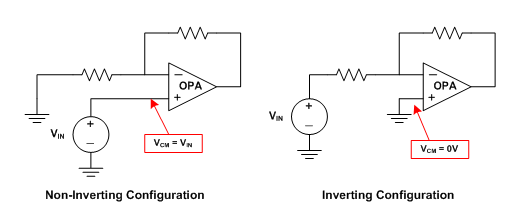Figure 1: Shows the common-mode voltage for the inverting and non-inverting op amp configuration

So then what is CMRR? The technical definition is the ratio of differential gain to common mode gain, but this doesn’t tell us much for a practical application. The common-mode input voltage affects the bias point of the input differential pair. Because of the inherent mismatches in the input circuitry, changing the bias point changes the input offset voltage (VOS), which, in turn, changes the output voltage. In other words, as you change your common-mode voltage you will see a change in input offset voltage. CMRR tells you how much that change will be.

CMRR is measured by changing the common-mode voltage and measuring the variation in offset voltage. Figure 2 shows a simple representation of the additive input offset voltage seen from changing the common-mode voltage. There are also offset errors associated with changing the supply voltage and the other external factors such as temperature and electromagnetic interference.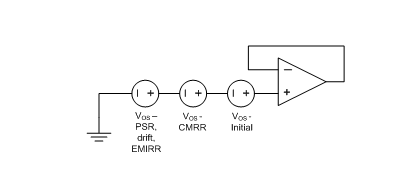Figure 2: representation of CMRR induced offset error

CMRR is expressed in decibels but for practical purposes is translated to microvolts per volt. The equations below show how to calculate how much error is seen in your application. The change in common-mode and input offset voltage is with respect to the nominal value specified in the datasheet,  usually mid-supply.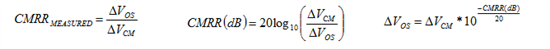CMRR also degrades with frequency, so how much error you see will be dependent on the frequency of your common-mode signal. Figure 3 shows an example of the CMRR vs. Frequency curve for 3 typical amplifiers, the OPA171, the UA741 and the OPA188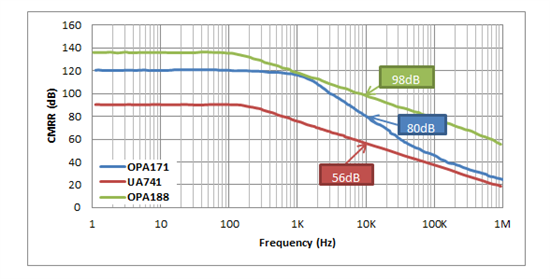Figure 3: CMRR vs. Frequency for 3 op amps

What does all this mean for your application? It depends on how you’re using the amplifier. For a quick example refer to figure 4 below. As you can see the CMRR of an amplifier depends on the frequency of interest and will cause an offset voltage variation that could have a large impact on the signal you are trying to refer to.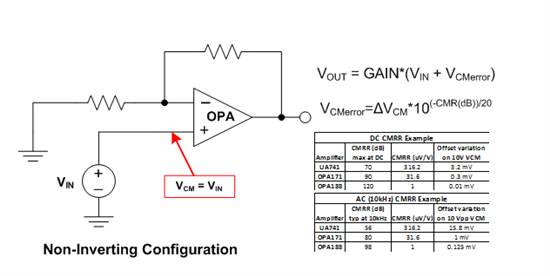Figure 4: CMRR error example

If you found this blog helpful, check out part 2 and 3 on instrumentation amplifiers and Instrumentation amplifier topologies. Thanks for reading!

• Will be great if along with formula a real example with actual numbers. Also for DC Vin also there is error right. Is there a graph for DC  Vin and Error.

• May I know how to measure this CMRR in TI lab or Ti fab.? thanks.

• Kevin Jerome, one of the assumptions about an ideal op amp is that the voltage at both the positive and negative inputs is the same.

• Hi Matthew.

Can you explain how the input signal become the common-mode signal in the non-inverting configuration ??

Regards